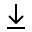MathBin Distance Member

Wikipedia says distance is a numerical description of how far apart objects are. I think length better fits this description, but that's just me. In fact, latter on the same page it states that in the physical sciences and engineering, when one speaks of "units of length", the word "length" is synonymous with "distance". Okay.

There is a saying that goes "The shortest path between two points is a straight line." In driving terms, if I drive my car on a straight road from point A to point B, my car's odometer will display the distance between the two points. So length and distance are the same, right?

What if I drive the same stretch of road, but somewhere I put my car in reverse and travel some distance backwards, then put the car into drive and moved forward to my destination? Now the odometer indicates I travelled farther than my first trip. So there is a difference between distance and length. Here is my distinction between the two, where the path is a line segment from point A to point B:

• Distance is how far one (object, particle, etc.) travels from point A to point B.
• Length is the distance measured between point A and point B.

Length can never be a negative value. Same for distance - you can travel backwards, but you cannot move back in time to obtain a negative distance. And the more I travel (forward or backward), the more distance (mileage) I accumulated. Length will always be less than or equal to distance.

Using my terminology, the Distance formula gives the length of the straight line between two points in any dimension. So we will concede that if there is only forward motion along a straight line from point A to point B, then distance and length are the same. At least they correctly named the Arc Length formula.

Bins in Two Dimensions Bins in Three Dimensions